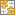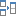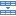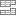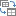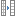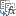# Excel ADDRESS function with formula examples

The tutorial gives a brief introduction to the ADDRESS function syntax and shows how to use it to return an Excel cell address and more.

To create a cell reference in Excel, you can type the column and row coordinates manually. Alternatively, you can get an Excel cell address from the row and column numbers supplied to the ADDRESS function. Almost pointless on its own, in combination with other functions this technique can be the only solution in situations when it is not possible to refer to a cell directly.

## Excel ADDRESS function - syntax and basic uses

The ADDRESS function is designed to get a cell address in Excel based on the specified row and column numbers. A cell address is returned as a text string, not an actual reference.

The syntax of the ADDRESS function is as follows:

The first two arguments are required:

row_num - the row number to use in the cell reference.

column_num - the column number to build the cell reference.

The last three arguments, which specify the cell reference format, are optional:

abs_num - the reference type, absolute or relative. It can take any of the below numbers; the default is absolute.

• 1 or omitted - absolute cell reference like \$A\$1
• 2 - mixed reference: relative column and absolute row like A\$1
• 3 - mixed reference: absolute column and relative row like \$A1
• 4 - relative cell reference such as A1

a1 - the reference style, A1 or R1C1. If omitted, the default A1 style is used.

• 1 or TRUE or omitted - returns the cell address in the A1 reference style where columns are letters and rows are numbers.
• 0 or FALSE - returns the cell address in the R1C1 reference style where rows and columns are represented by numbers.

sheet_text - the name of the worksheet to include in the external reference. The sheet name should be supplied as a text string and enclosed in quotation marks, e.g. "Sheet2". If omitted, no worksheet name is used, and the address defaults to the current sheet.

For example:

`=ADDRESS(1,1)` - returns the address of the first cell (i.e. the cell at the intersection of the first row and first column) as an absolute cell reference \$A\$1.

`=ADDRESS(1,1,4)` - returns the address of the first cell as a relative cell reference A1.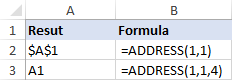In the following table, you will find a few more reference types that can be returned by ADDRESS formulas.

 Formula Result Description =ADDRESS(1,2) \$B\$1 Absolute cell reference =ADDRESS(1,2,4) B1 Relative cell reference =ADDRESS(1,2,2) B\$1 Relative column and absolute row =ADDRESS(1,2,3) \$B1 Absolute column and relative row =ADDRESS(1,2,1,FALSE) R1C2 Absolute reference in the R1C1 style =ADDRESS(1,2,4,FALSE) RC Relative reference in the R1C1 style =ADDRESS(1,2,1,,"Sheet2") Sheet2!\$B\$1 Absolute reference to another sheet =ADDRESS(1,2,4,,"Sheet2") Sheet2!B1 Relative reference to another sheet

## How to use ADDRESS function in Excel - formula examples

The below examples show how to use the ADDRESS function inside bigger formulas to accomplish more difficult tasks.

### Return a cell value in a given row and column

If your goal is to get a value from a specific cell based on its row and column numbers, use the ADDRESS function together with INDIRECT:

The ADDRESS function outputs the cell address as text. The INDIRECT function turns that text into a normal reference and returns the value from the corresponding cell.

For example, to get a cell value based on the row number in E1 and the column number in E2, use this formula:

`=INDIRECT(ADDRESS(E1,E2))`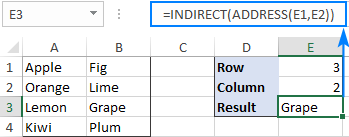### Get the address of a cell with the highest or lowest value

In this example, we'll first find the highest and lowest values in the range B2:B7 by using the MAX and MIN functions and output those values into special cells:

Cell E2: `=MAX(B2:B7)`

Cell F2: `=MIN(B2:B7)`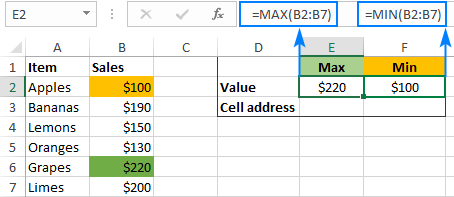And then, we'll use ADDRESS in combination with the MATCH function to get the cell addresses.

Cell with the max value:

`=ADDRESS(MATCH(E2,B:B,0), COLUMN(B2))`

Cell with the min value:

`=ADDRESS(MATCH(F2,B:B,0), COLUMN(B2))`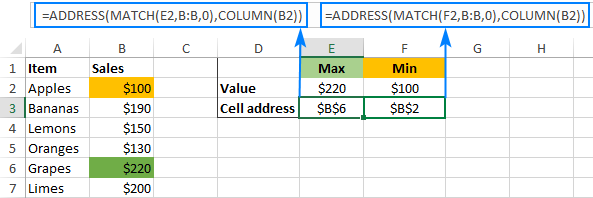In case you do not want the highest and lowest values in separate cells, you can nest the MAX/MIN function in the first argument of MATCH. For example:

Cell with the highest value:

`=ADDRESS(MATCH(MAX(B2:B7),B:B,0), COLUMN(B2))`

Cell with the lowest value:

`=ADDRESS(MATCH(MIN(B2:B7),B:B,0), COLUMN(B2))`

#### How these formulas work

To find the row number, you use the MATCH(lookup_value, lookup_array, [match_type]) function that returns a relative position of lookup_value in lookup_array. In our formula, the lookup value is the number returned by the MAX or MIN function, and the lookup array is the entire column. Consequently, a relative position of the lookup value in the array exactly matches the row number on the sheet.

To find the column number, you use the COLUM function. Of course, nothing prevents you from typing the number directly in the formula, but COLUMN saves the trouble of manual counting in case the target column is in the middle of the sheet.

### Get a column letter from a column number

To turn any given number into a column letter, use the ADDRESS function inside of SUBSTITUTE:

As an example, let's find the column letter corresponding to the number in A2:

`=SUBSTITUTE(ADDRESS(1,A2,4),"1","")`

Looking at the results below, we can say that the first column on the sheet is A, which is obvious; the 10th column is J, the 50th column is AX, and the 100th column is CV: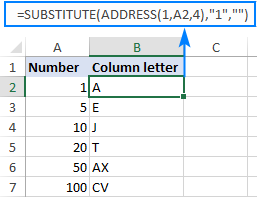#### How this formula works

For starters, set up the ADDRESS function to return a relative reference to the first cell in the target column:

• For the row number, use 1.
• For the column number, supply the reference to the cell containing the number, A2 in our example.
• For the abs_num argument, enter 4.

As the result, ADDRESS(1,A2,4) would return A1.

To get rid of the row coordinate, wrap the above formula in the SUBSTITUTE function and replace "1" with an empty string (""). Done!

### Get the address of a named range

To find the address of a named range in Excel, you will first need to obtain the first and last cell references, and then join them together.

#### How to get address of the first cell in a range

To return a reference to the first cell in a named range, use this generic formula:

Assuming the range is named "Sales", the real formula goes as follows:

`=ADDRESS(ROW(Sales), COLUMN(Sales))`

And returns the address of the upper left cell in the range: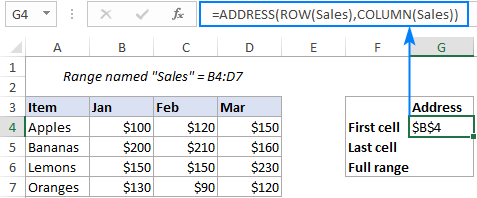In this formula, the ROW and COLUMN functions return an array of all the row and column numbers in the range, respectively. Based on those numbers, the ADDRESS function builds an array of cell addresses. But because the formula is entered in a single cell, only the first item of the array is displayed, which corresponds to the first cell in the range.

#### How to get address of the last cell in a range

To find the address of the last cell in a named range, use this generic formula:

Applied to our range named "Sales", the formula takes the following shape:

`=ADDRESS(ROW(Sales) + ROWS(Sales)-1, COLUMN(Sales) + COLUMNS(Sales)-1)`

And returns the reference to the bottom right cell of the range: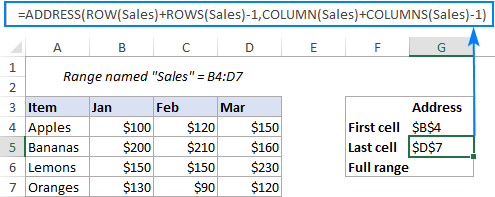This time, we need a bit more complex calculations to work out the row number. Like in the previous example, the ROW function gives us an array of all the row numbers in the range, {4;5;6;7} in our case. We need to "shift" these numbers by the total row count minus 1, so that the first item in the array becomes the last row number. To find the total row count, we employ the ROWS function and subtract 1 from its result: (4-1=3). Then, we add 3 to each element of the initial array to do the required shift: {4;5;6;7} + 3 = {7;8;9;10}.

The column number is calculated in a similar manner: {2,3,4}+3-1 = {4,5,6}

From the above arrays of row and column numbers, the ADDRESS function assembles an array of cell addresses, but returns only the first one corresponding to the last cell in the range.

The same result can also be achieved by picking the maximum values from the arrays of the row and column numbers. However, this only works in an array formula, which requires pressing Ctrl + Shift + Enter to be completed correctly:

`=ADDRESS(MAX(ROW(Sales)), MAX(COLUMN(Sales)))`

#### How to get full address of a named range

To return the complete address of a named range, you just need to concatenate the two formulas from the previous examples and insert the range operator (:) in-between.

To make it work for our sample data set, we replace the generic "range" with the real range name "Sales":

`=ADDRESS(ROW(Sales), COLUMN(Sales)) & ":" & ADDRESS(ROW(Sales) + ROWS(Sales)-1, COLUMN(Sales) + COLUMNS(Sales)-1)`

And get the complete range address as an absolute reference \$B\$4:\$D\$7: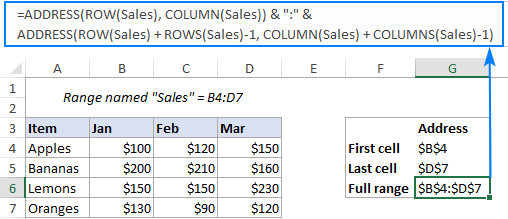To return the range address as a relative reference (without the \$ sign, like B4:D7), set the abs_num argument in both ADDRESS functions to 4:

`=ADDRESS(ROW(Sales), COLUMN(Sales), 4) & ":" & ADDRESS(ROW(Sales) + ROWS(Sales)-1, COLUMN(Sales) + COLUMNS(Sales)-1, 4)`

Naturally, the same changes can be made in individual formulas for the first and last cell, and the result will look similar to this: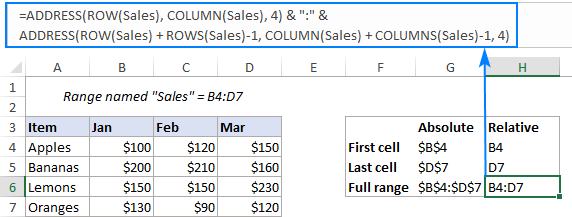That's how you return a cell address in Excel. To have closer look at all the formulas discussed in this tutorial, you are welcome to download our Excel ADDRESS Function Sample Workbook. I thank you for reading and hope to see you on our blog next week!

### 15 responses to "Excel ADDRESS function with formula examples"

1.ANILKUMAR K N says:

I’m trying to find the address of the First five row numbers in a given column where continuously the cell values are >= X, There can be multiple instances where we can find the continuous rows where cell values are >=X, but I need to find the first occurrence only.

For example in column A, continuously values in the 5 rows i.e., A6 A7 A8 A9 A10 are >=x and also in row number A15 A16 A17 A18 A19, etc.
The row# A6 to A10 is the first occurrence (first five consecutive values) and I need the address of the row numbers as output i.e., A6: A10

2.texugo indy says:

Hi Svetlana Cheusheva. I have this formula:
That returns:
'[Book1 (version 1).xlsb]Sheet2'!\$D\$1
How to show not the file name?

3.Anurag says:

is there any function in excel that finds the requied value in a range and returns Left most column value and top most row value in that range for the found cell.

4.Anurag says:

is there any function in excel that finds the requied value in a range and returns Left most column value and top most row value in that range for the found cell.

5.Remy says:

I have such SUMIFS formula working fine:
=SUMIFS('Taches Dev'!V3:V\$1000,'Taches Dev'!B\$3:B\$1000,A2,'Taches Dev'!C\$3:C\$1000,L\$1)
where
'Taches Dev'!V3:V\$1000 is my sum range.

In another 2 cells, I have formulas returning the adresses of the two bounds of my range
=ADRESSE(3;EQUIV(M1;'Taches Dev'!A2:AI2;0);;;"Taches Dev") --> Taches Dev'!V3

I'd like to use directly these 2 formulas into the SUMIFS function for determining my sum range.

But I'm getting an error.
Any idea?

•Alexander Trifuntov (Ablebits.com Team) says:

Hello Remy!
To specify a range of cells, you can use the following formula:

INDIRECT(B21&":"&B23)

I hope this will help, otherwise please do not hesitate to contact me anytime.

6.Duc says:

I am trying to get the string from cell address.
something that does this: =SomeFormula(A1) returns A1 as string.

•Alexander Trifuntov (Ablebits.com Team) says:

Hello!
If I understand your task correctly, the following formula should work for you:

7.GIRISH GANGAJALIWALE says:

=ADDRESS(MATCH('ROOM1'!\$Q\$11'ROLL NUMBERS'!A:A,0)+1,1) NOT GETTING REFRENCE OF SECOND SHEET FROM SHEET 1

•Alexander Trifuntov (Ablebits.com Team) says:

Hello!
I cannot validate the formula on your data. But something like this will work for you

Hope this is what you need.

8.rob says:

Hi, I am trying to increment the row number of the value of a cell. This value references another workbook.
The formula in cell A1 which gives me the absolute address from the other workbook is =CELL("ADDRESS",INDEX('[WORKBOOKNAME.XLS]WORKSHEETNAME'!\$A:\$A,MATCH("*WA(*",'[WORKBOOKNAME.XLS]WORKSHEETNAME'!\$A:\$A,0)))
The value of this results in:
'[WORKBOOKNAME.XLS]WORKSHEETNAME'!\$A:\$251
I now want to use this address in another formula but increment by 1 for each row it is used in
Is this possible, many thanks

•Alexander Trifuntov (Ablebits.com Team) says:

Hello!
\$A:\$251 - this link is not possible.
Write down exactly what you are getting now and what you want to get when you move to the next line. Describe your problem in more detail.

•Rob says:

Ultimately I want to use the cell address of a cell from another workbook.
In order to do this I search a column in the different workbook for a matching string and return its cell address;
this works and gives me a result in cell A1 of...
'[WORKBOOKNAME.XLS]WORKSHEETNAME'!\$A:\$251
I then want to use this cell address in another formula to retrieve the value of the cells and offset cells in the other workbook.

I don't want to use VBA.

9.Stephanie says:

I am trying to figure out which functions to use to find ”number of people attending”, from a column with blank cells and zeros. However I have the cost of each invitation, tax, and the amount it adds up to. Help!

•Alexander Trifuntov (Ablebits.com Team) says:

Hello!
Your question is not related to using the ADDRESS function. I recommend that you study this guide on counting the number of values and, if necessary, ask your question there.

Ultimate Suite for Microsoft Excel